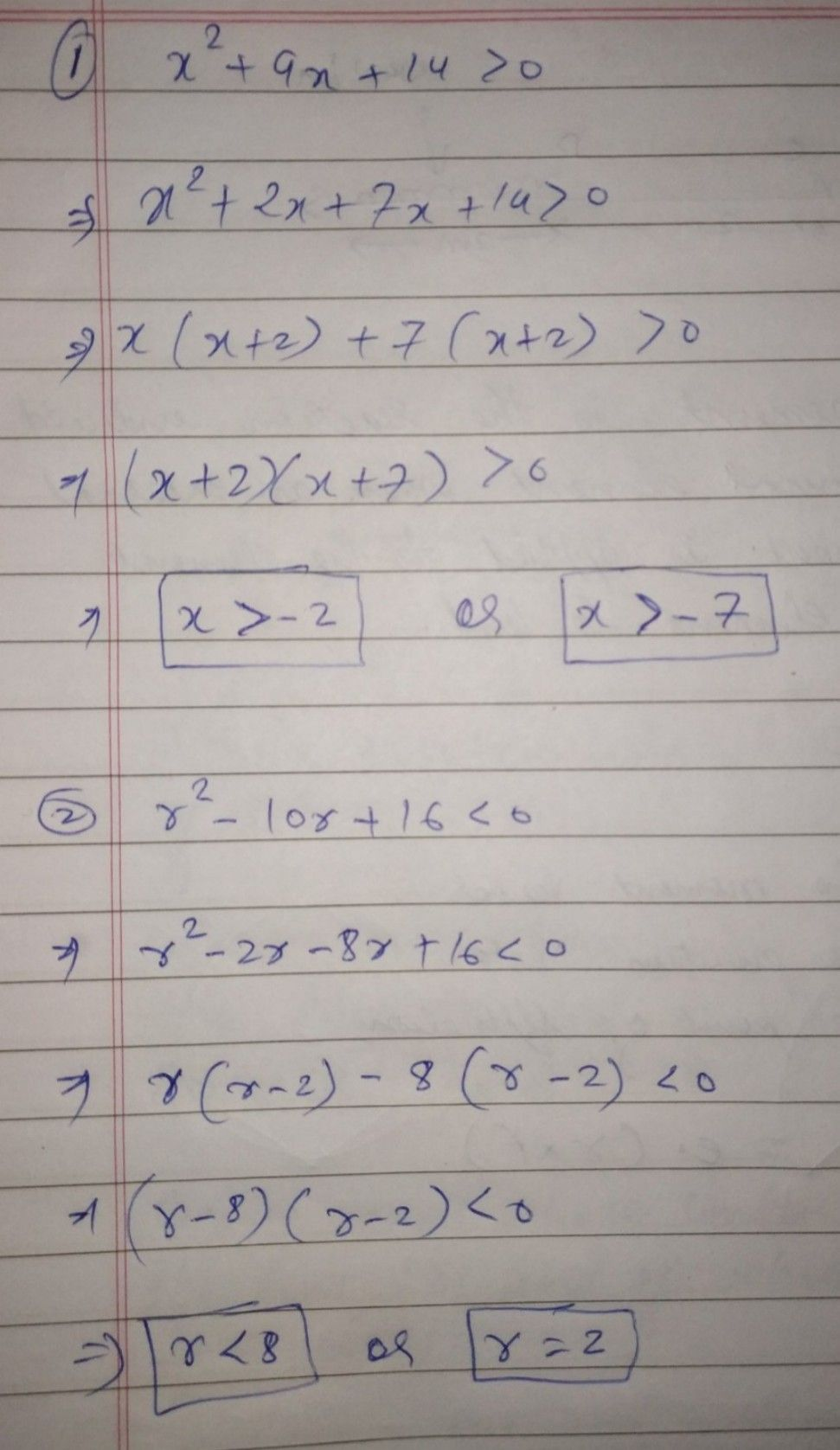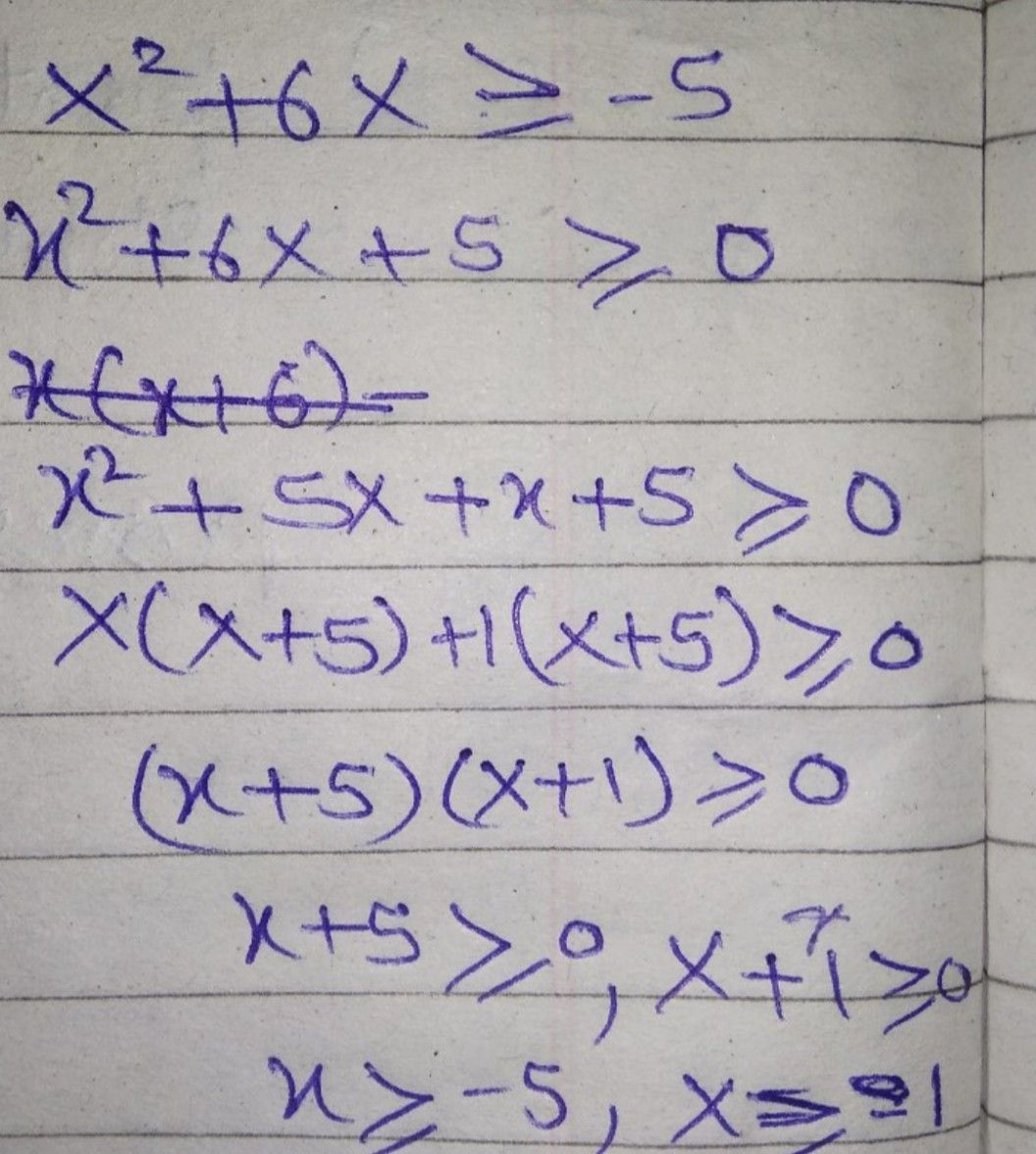Symbol
Problem$Direct/0ns$ Find the solution set of each of the following quadratic inequalities then graph. Answer the questions that follow. 1. $x^{2}+9x+14>0$ 6. $2t^{2}+11t+12<0$ 2. $r^{2}-10r+16<0$ $7$ $3x^{2}-8x+5\geq 0$ 3. $x^{2}+6x\geq -5$ 8. $4p^{2}\leq 1$ $4$ $m^{2}-7m\leq 10$ $9$ $2x^{2}-3x-14>0$ 5. $x^{2}-5x-14>0$ $10.3q^{2}+2q\geq 5$ $vestions$ a. How did you find the solution set of each inequality? b. What mathematics concepts or principles did you apply to $comθ$ up with the solution set of each mathematical sentence? C. How did you graph the solution set of each inequality? d. How would you describe the graph of the solution set of a Quadratic Inequality? e. How many solutions does each inequality have? f. Are the solution(s) of each inequality real numbers? Why? $9$ Is it possible for a Quadratic Inequality not to have a real solution? Justify your answer by giving a particular example. $-$